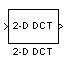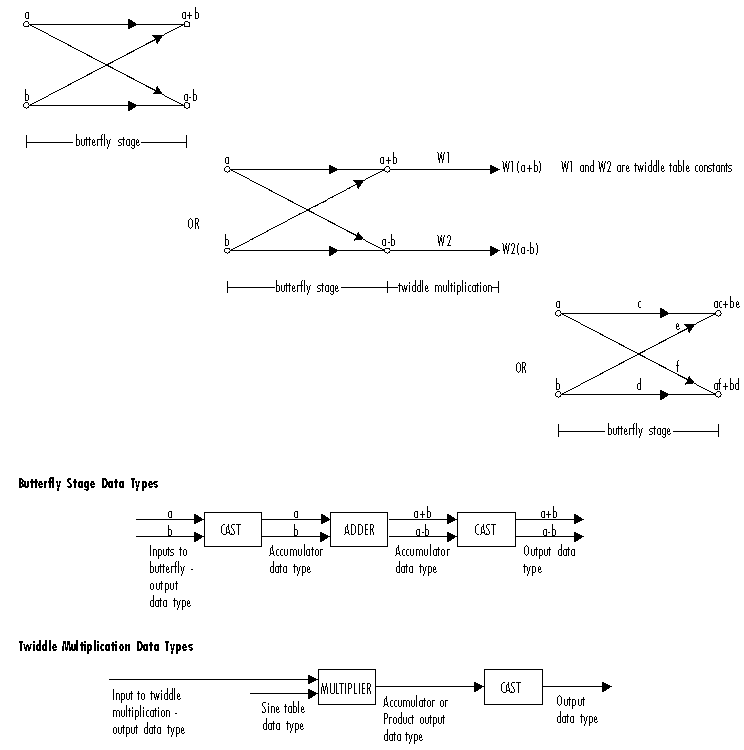Documentation

# 2-D DCT

Compute 2-D discrete cosine transform (DCT)

## Library

Transforms

`visiontransforms`## Description

The 2-D DCT block calculates the two-dimensional discrete cosine transform of the input signal. The equation for the two-dimensional DCT iswhere $C\left(m\right),C\left(n\right)=1/\sqrt{2}$ for $m,n=0$ and $C\left(m\right),C\left(n\right)=1$ otherwise.

The number of rows and columns of the input signal must be powers of two. The output of this block has dimensions the same dimensions as the input.

PortInput/OutputSupported Data TypesComplex Values Supported

Input

Vector or matrix of intensity values

• Double-precision floating point

• Single-precision floating point

• Fixed point

• 8-, 16-, 32-bit signed integer

• 8-, 16-, 32-bit unsigned integer

No

Output

2-D DCT of the input

Same as Input port

No

If the data type of the input signal is floating point, the output of the block is the same data type.

Use the Sine and cosine computation parameter to specify how the block computes the sine and cosine terms in the DCT algorithm. If you select `Trigonometric fcn`, the block computes the sine and cosine values during the simulation. If you select `Table lookup`, the block computes and stores the trigonometric values before the simulation starts. In this case, the block requires extra memory.

### Fixed-Point Data Types

The following diagram shows the data types used in the 2-D DCT block for fixed-point signals. Inputs are first cast to the output data type and stored in the output buffer. Each butterfly stage processes signals in the accumulator data type, with the final output of the butterfly being cast back into the output data type.The output of the multiplier is in the product output data type when at least one of the inputs to the multiplier is real. When both inputs to the multiplier are complex, the result of the multiplication is in the accumulator data type. For details on the complex multiplication performed, refer to Multiplication Data Types. You can set the sine table, product output, accumulator, and output data types in the block mask as discussed in the next section.

## Parameters

Sine and cosine computation

Specify how the block computes the sine and cosine terms in the DCT algorithm. If you select `Trigonometric fcn`, the block computes the sine and cosine values during the simulation. If you select `Table lookup`, the block computes and stores the trigonometric values before the simulation starts. In this case, the block requires extra memory.

Rounding mode

Select the Rounding Modes for fixed-point operations. The sine table values do not obey this parameter; they always round to `Nearest`.

Saturate on integer overflow

Select the overflow mode for fixed-point operations. See Precision and Range. The sine table values do not obey this parameter; instead, they are always saturated.

Sine table data type

Choose how you specify the word length of the values of the sine table. The fraction length of the sine table values always equals the word length minus one. You can set this parameter to:

• A rule that inherits a data type, for example, ```Inherit: Same word length as input```

• An expression that evaluates to a valid data type, for example, `fixdt(1,16)`

The sine table values do not obey the Rounding mode and Saturate on integer overflow parameters; instead, they are always saturated and rounded to `Nearest`.

Product output data type

Specify the product output data type. See Fixed-Point Data Types and Multiplication Data Types for illustrations depicting the use of the product output data type in this block. You can set this parameter to:

• A rule that inherits a data type, for example, ```Inherit: Inherit via internal rule```

• An expression that evaluates to a valid data type, for example, `fixdt(1,16,0)`

Click the buttonto display the Data Type Assistant, which helps you set the Product output data type parameter.

See Specify Data Types Using Data Type Assistant (Simulink) for more information.

Accumulator data type

Specify the accumulator data type. See Fixed-Point Data Types for illustrations depicting the use of the accumulator data type in this block. You can set this parameter to:

• A rule that inherits a data type, for example, ```Inherit: Inherit via internal rule```

• An expression that evaluates to a valid data type, for example, `fixdt(1,16,0)`

Click the buttonto display the Data Type Assistant, which helps you set the Accumulator data type parameter.

See Specify Data Types Using Data Type Assistant (Simulink) for more information.

Output data type

Specify the output data type. See Fixed-Point Data Types for illustrations depicting the use of the output data type in this block. You can set this parameter to:

• A rule that inherits a data type, for example, ```Inherit: Inherit via internal rule```.

When you select `Inherit: Inherit via internal rule`, the block calculates the output word length and fraction length automatically. The internal rule first calculates an ideal output word length and fraction length using the following equations:

`$W{L}_{idealoutput}=W{L}_{input}+floor\left({\mathrm{log}}_{2}\left(DCTlength-1\right)\right)+1$`
`$F{L}_{idealoutput}=F{L}_{input}$`

Using these ideal results, the internal rule then selects word lengths and fraction lengths that are appropriate for your hardware. For more information, see Inherit via Internal Rule.

• An expression that evaluates to a valid data type, for example, `fixdt(1,16,0)`

Click the buttonto display the Data Type Assistant, which helps you set the Output data type parameter.

See Specify Data Types Using Data Type Assistant (Simulink) for more information.

Lock scaling against changes by the autoscaling tool

Select this parameter to prevent any fixed-point scaling you specify in this block mask from being overridden by the autoscaling tool in the Fixed-Point Tool. For more information, see `fxptdlg`, a reference page on the Fixed-Point Tool in the Simulink® documentation.

Lock data type settings against change by the fixed-point tools

Select this parameter to prevent the fixed-point tools from overriding the data types you specify on the block mask. For more information, see `fxptdlg`, a reference page on the Fixed-Point Tool in the Simulink documentation.

## References

 Chen, W.H, C.H. Smith, and S.C. Fralick, “A fast computational algorithm for the discrete cosine transform,” IEEE Trans. Commun., vol. COM-25, pp. 1004-1009. 1977.

 Wang, Z. “Fast algorithms for the discrete W transform and for the discrete Fourier transform,” IEEE Trans. Acoust., Speech, Signal Processing, vol. ASSP-32, pp. 803-816, Aug. 1984.

 2-D IDCT Computer Vision Toolbox™ software 2-D FFT Computer Vision Toolbox software 2-D IFFT Computer Vision Toolbox software